Anúncio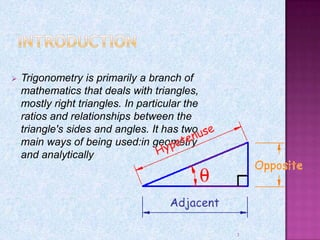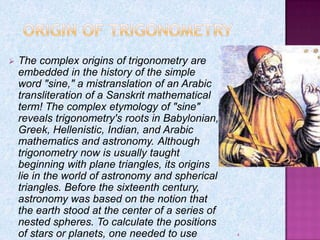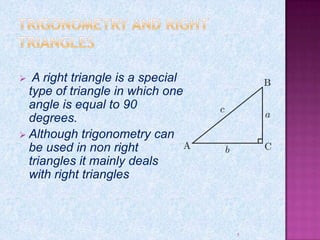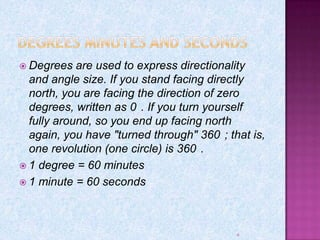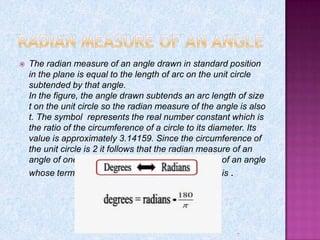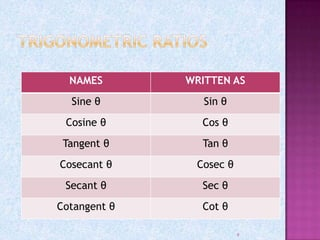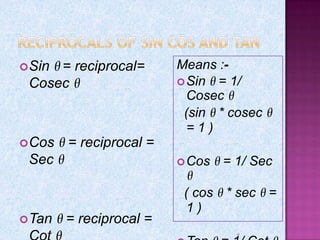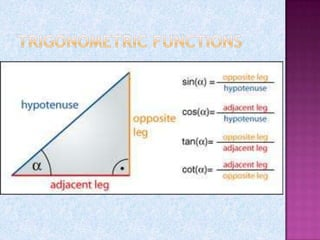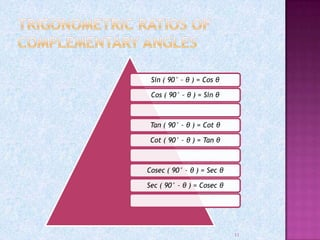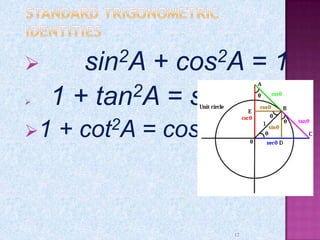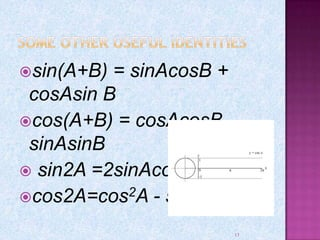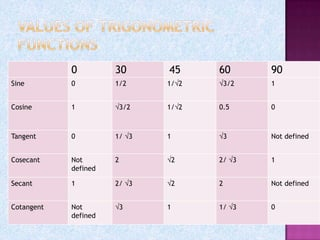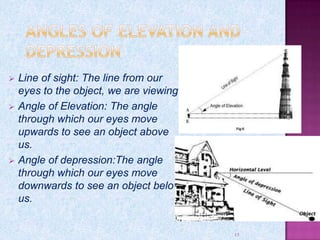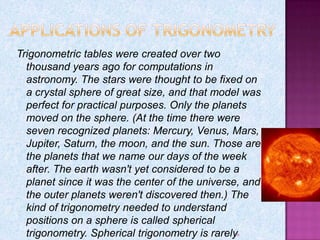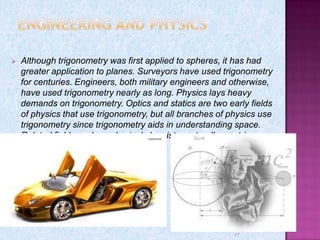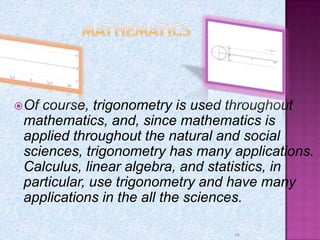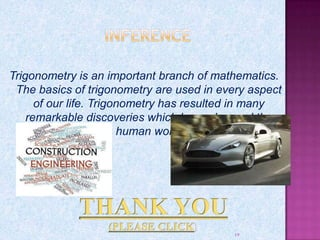1 de 19
Anúncio

### trigonometry-130721043143-phpapp02.pdf

1. Kartikeya Pandey 10th Class – Section: A Cambridge School Indirapuram 1
2. I would like to express my special thanks of gratitude to my teacher Ms Meha Bhargava as well as our principal Ms Jasleen Kaur who gave me the golden opportunity to do this wonderful project on the topic Trigonometry, which also helped me in doing a lot of Research and I came to know about so many new things I am really thankful to them. Secondly I would also like to thank my parents and friends who helped me a lot in finishing this project within the limited time. 2
3.  Trigonometry is primarily a branch of mathematics that deals with triangles, mostly right triangles. In particular the ratios and relationships between the triangle's sides and angles. It has two main ways of being used:in geometry and analytically 3
4.  The complex origins of trigonometry are embedded in the history of the simple word "sine," a mistranslation of an Arabic transliteration of a Sanskrit mathematical term! The complex etymology of "sine" reveals trigonometry's roots in Babylonian, Greek, Hellenistic, Indian, and Arabic mathematics and astronomy. Although trigonometry now is usually taught beginning with plane triangles, its origins lie in the world of astronomy and spherical triangles. Before the sixteenth century, astronomy was based on the notion that the earth stood at the center of a series of nested spheres. To calculate the positions of stars or planets, one needed to use 4
5.  A right triangle is a special type of triangle in which one angle is equal to 90 degrees.  Although trigonometry can be used in non right triangles it mainly deals with right triangles 5
6.  Degrees are used to express directionality and angle size. If you stand facing directly north, you are facing the direction of zero degrees, written as 0 . If you turn yourself fully around, so you end up facing north again, you have "turned through" 360 ; that is, one revolution (one circle) is 360 .  1 degree = 60 minutes  1 minute = 60 seconds 6
7.  The radian measure of an angle drawn in standard position in the plane is equal to the length of arc on the unit circle subtended by that angle. In the figure, the angle drawn subtends an arc length of size t on the unit circle so the radian measure of the angle is also t. The symbol represents the real number constant which is the ratio of the circumference of a circle to its diameter. Its value is approximately 3.14159. Since the circumference of the unit circle is 2 it follows that the radian measure of an angle of one revolution is 2. The radian measure of an angle whose terminal side is along the negative x -axis is . 7
8. 8 NAMES WRITTEN AS Sine θ Sin θ Cosine θ Cos θ Tangent θ Tan θ Cosecant θ Cosec θ Secant θ Sec θ Cotangent θ Cot θ
9. 9 Sin θ = reciprocal= Cosec θ Cos θ = reciprocal = Sec θ Tan θ = reciprocal = Means :- Sin θ = 1/ Cosec θ (sin θ * cosec θ = 1 ) Cos θ = 1/ Sec θ ( cos θ * sec θ = 1 )
10. Sin ( 90° – θ ) = Cos θ Cos ( 90° – θ ) = Sin θ Tan ( 90° – θ ) = Cot θ Cot ( 90° – θ ) = Tan θ Cosec ( 90° – θ ) = Sec θ Sec ( 90° – θ ) = Cosec θ 11
11.  sin2A + cos2A = 1  1 + tan2A = sec2A 1 + cot2A = cosec2A 12
12. sin(A+B) = sinAcosB + cosAsin B cos(A+B) = cosAcosB – sinAsinB  sin2A =2sinAcosA cos2A=cos2A - sin2A 13
13. 14 0 30 45 60 90 Sine 0 1/2 1/ 2 3/2 1 Cosine 1 3/2 1/ 2 0.5 0 Tangent 0 1/ 3 1 3 Not defined Cosecant Not defined 2 2 2/ 3 1 Secant 1 2/ 3 2 2 Not defined Cotangent Not defined 3 1 1/ 3 0
14.  Line of sight: The line from our eyes to the object, we are viewing.  Angle of Elevation: The angle through which our eyes move upwards to see an object above us.  Angle of depression:The angle through which our eyes move downwards to see an object below us. 15
15. 16 Trigonometric tables were created over two thousand years ago for computations in astronomy. The stars were thought to be fixed on a crystal sphere of great size, and that model was perfect for practical purposes. Only the planets moved on the sphere. (At the time there were seven recognized planets: Mercury, Venus, Mars, Jupiter, Saturn, the moon, and the sun. Those are the planets that we name our days of the week after. The earth wasn't yet considered to be a planet since it was the center of the universe, and the outer planets weren't discovered then.) The kind of trigonometry needed to understand positions on a sphere is called spherical trigonometry. Spherical trigonometry is rarely
16.  Although trigonometry was first applied to spheres, it has had greater application to planes. Surveyors have used trigonometry for centuries. Engineers, both military engineers and otherwise, have used trigonometry nearly as long. Physics lays heavy demands on trigonometry. Optics and statics are two early fields of physics that use trigonometry, but all branches of physics use trigonometry since trigonometry aids in understanding space. Related fields such as physical chemistry naturally use trig. 17
17. Of course, trigonometry is used throughout mathematics, and, since mathematics is applied throughout the natural and social sciences, trigonometry has many applications. Calculus, linear algebra, and statistics, in particular, use trigonometry and have many applications in the all the sciences. 18
18. Trigonometry is an important branch of mathematics. The basics of trigonometry are used in every aspect of our life. Trigonometry has resulted in many remarkable discoveries which have changed the human world 19
Anúncio